##### PostgreSQL TO_CHAR Function With Practical Examples

Postgres offers a built-in function named TO_CHAR() that converts any data type, such as an integer, interval, timestamp, date, etc., to a string. The TO_CHAR() function utilizes a format mask to convert the input value to a string. The format mask must be a valid number or date.

This blog post will show you how to use the TO_CHAR() function in PostgreSQL via practical examples. The below-mentioned concepts will be discussed in this write-up:

• How to Use TO_CHAR() Function in Postgres?
• How Do I Convert a Timestamp to a String in Postgres Via TO_CHAR() Function?
• How to Convert an Interval to a String in Postgres Via TO_CHAR() Function?
• How Do I Convert a Date Field to a String in Postgres Via TO_CHAR() Function?
• How Do I Convert an Integer Value to a String in Postgres Via TO_CHAR() Function?
• How Do I Convert a Numeric Value to a String in Postgres Via TO_CHAR() Function?

So, let’s begin!

How to Use TO_CHAR() Function in Postgres?

In Postgres, the TO_CHAR() function converts a date, number, interval, etc., to a string. To put the TO_CHAR() function into practice, you must follow the below-given syntax:

`TO_CHAR(exp, format);`

In the above syntax:

- exp represents an expression to be converted.
- The specified expression can be a date/time stamp, an integer, a number, a double precision, or an interval.
- The format represents a valid/permitted numeric or timestamp format based on which the given expression will be converted into a string.

Valid numeric and timestamp formats are explained in this document.

Example 1: How Do I Convert a Timestamp to a String in Postgres Via TO_CHAR() Function?

In the following example code, we are going to use the current timestamp as the first argument and a format as a second argument in the TO_CHAR() function.

`SELECT TO_CHAR(CURRENT_TIMESTAMP, 'YYYY-MM-DD HH24:MM:SS');`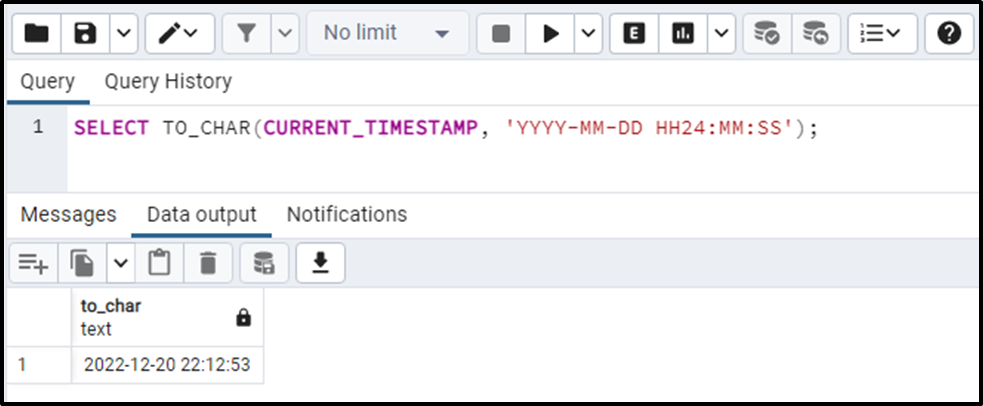On successful execution of the TO_CHAR() function, the current date and time will be converted into a string:

The output snippet shows that the specified timestamp was successfully converted into the string.

Example 2: How to Convert an Interval to a String in Postgres Via TO_CHAR() Function?

Let’s pass an interval as the first parameter and a valid format as the second parameter to the TO_CHAR() function:

`SELECT TO_CHAR(INTERVAL '5 year 6 months 12 days', 'YYYY/MM/DD');`

On successful execution, the TO_CHAR() function will retrieve a string in YYYY/MM/DD format: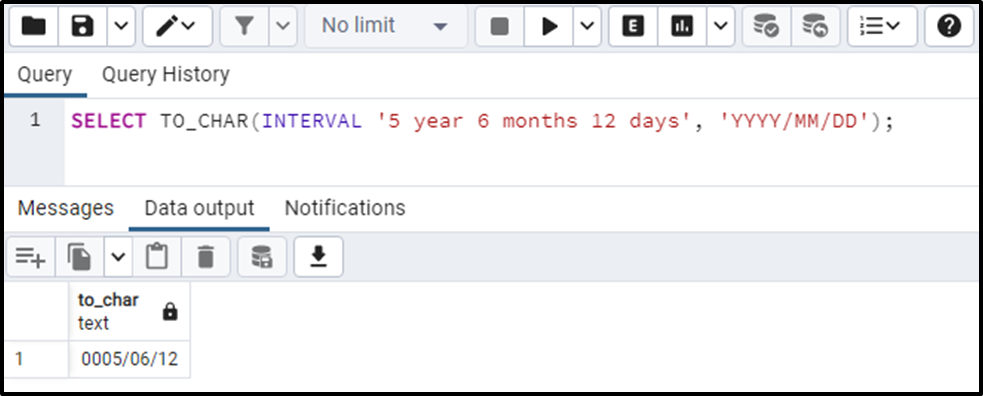The above snippet proves that the given interval has been successfully converted into a string using the TO_CHAR() function.

Example 3: How Do I Convert a Date Field to a String in Postgres Via TO_CHAR() Function?

To convert the current date into a string, let’s pass the current date and a valid format as arguments to the TO_CHAR() function.

`SELECT TO_CHAR(CURRENT_DATE, 'MON DD, YYYY');`

The above piece of code will convert the current date into a “MON DD, YYYY” format: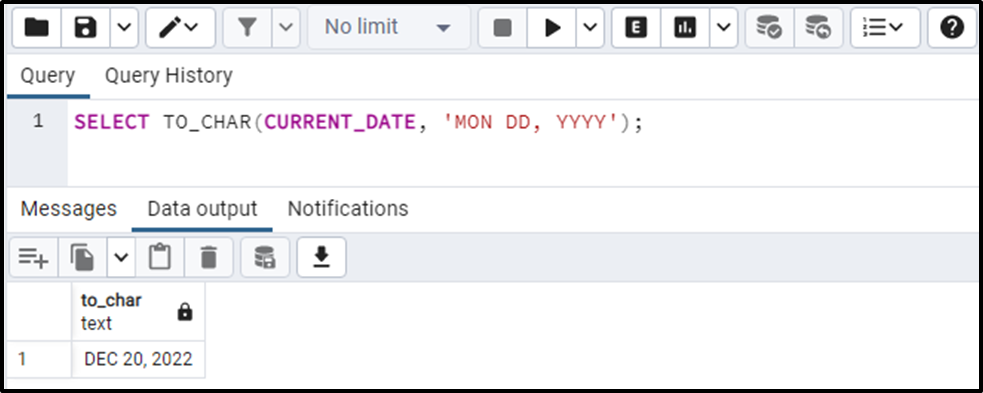The output authenticates the working of the TO_CHAR() function.

Example 4: How Do I Convert an Integer Value to a String in Postgres Via TO_CHAR() Function?

Let’s pass an integer and a valid numeric format to the TO_CHAR function to convert the input number into a string:

`SELECT TO_CHAR(572172, '999,999');`

The above code uses "9" to represent numeric values and commas to separate them: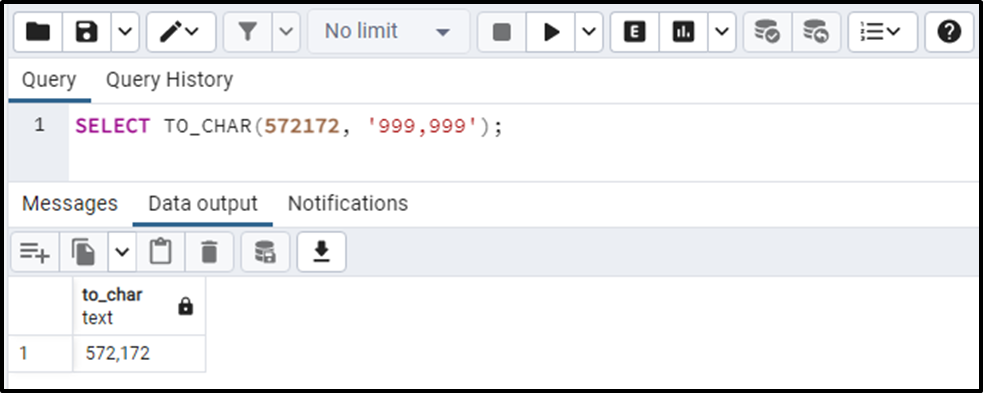The output snippet shows that the given integer has been converted into a string via the TO_CHAR() function.

Example 5: How to Convert a NUMERIC Value to a String in Postgres Via TO_CHAR() Function?

This example will teach you how to use the TO_CHAR() function to convert a numeric value to a string using a valid numeric format:

`SELECT TO_CHAR(572.172, '999D999');`

The “D” is a numeric format used to represent a decimal point: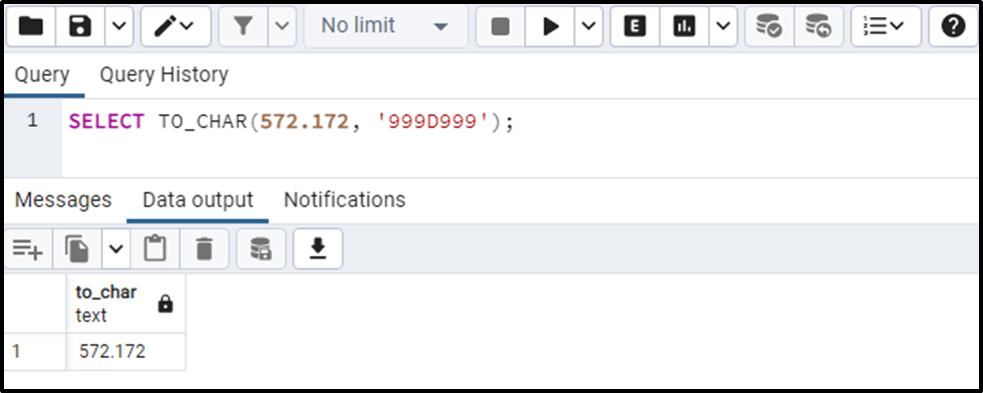The given numeric value has been successfully converted into a string via the TO_CHAR() function.

Example 6: How to Use the TO_CHAR() Function on Table’s Data?

We have created a sample table named “article_information” that contains the following data:

`SELECT * FROM article_infromation;`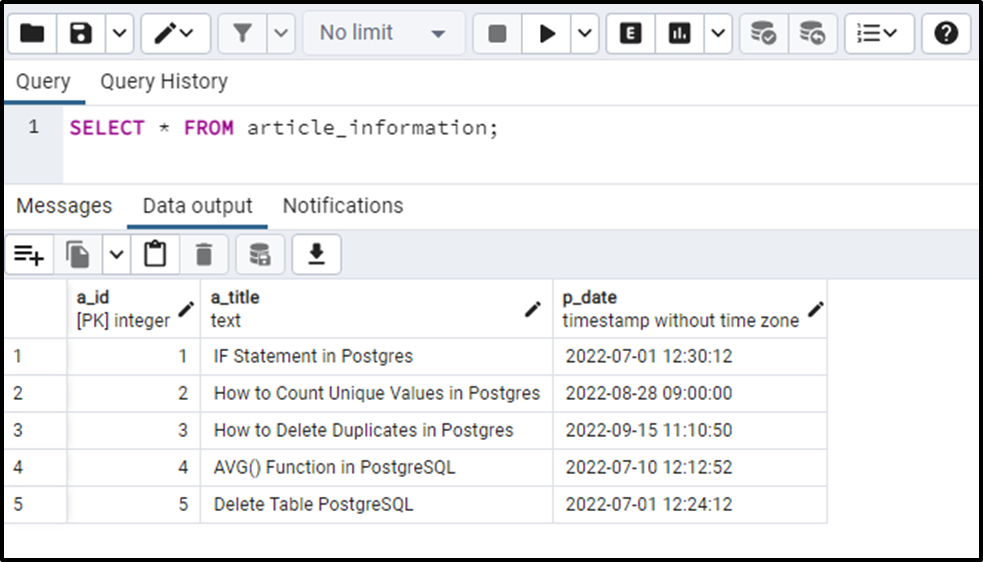We will use the TO_CHAR() function to convert the TIMESTAMP column to a string. After that, we will use the concatenation operator “||” to concatenate the “a_title” and “p_date” columns:

```SELECT a_title || ' Published on ' ||
TO_CHAR(p_date, 'YYYY/MM/DD') as article_info
FROM article_information;```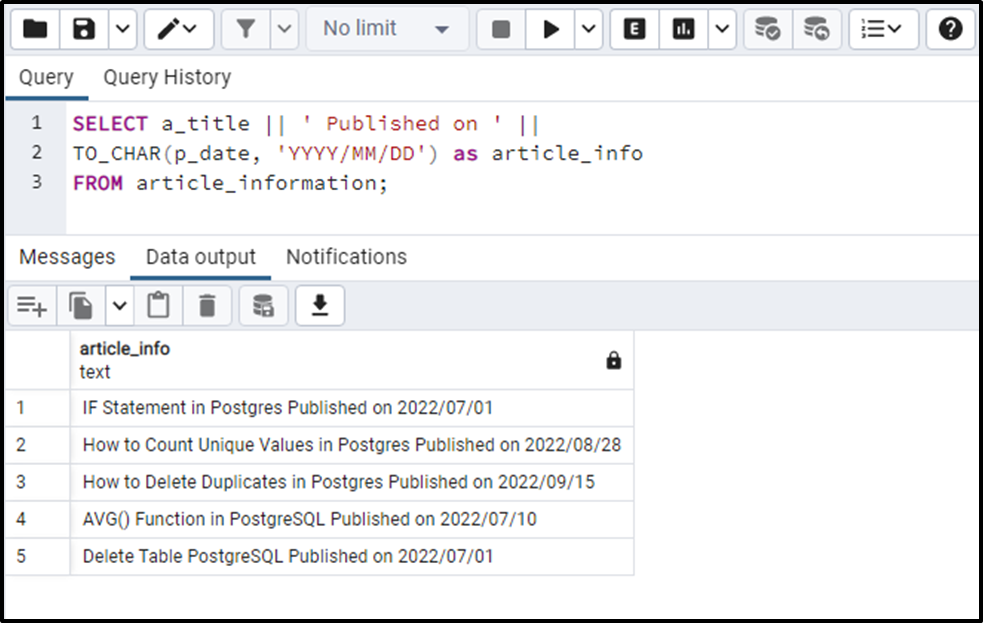The output snippet shows that the "p_date" column has been successfully converted to a string and concatenated with the "a_title" column.

That’s it from this blog!

Conclusion

In Postgres, a built-in function named TO_CHAR() is used to convert any data type, such as an integer, interval, timestamp, date, etc., to a string. The TO_CHAR() function utilizes a format mask to convert the input value to a string. The format mask must be a valid number or date. This Postgres blog presented various examples to explain the working of the TO_CHAR() function.